# SBI PO Prelims Reasoning Ability Questions 2021 (Day-02)

Dear Aspirants, Our IBPS Guide team is providing new series of Reasoning Questions for SBI PO 2021 Prelims. so the aspirants can practice it on a daily basis. These questions are framed by our skilled experts after understanding your needs thoroughly. Aspirants can practice these new series questions daily to familiarize with the exact exam pattern and make your preparation effective. Added to that we have given SBI PO Prelims free practice set pdf and SBI PO Book PDF for download.

## Start Quiz

Order and ranking

Directions (1-3): Answer the questions based on the information given below.

Seven persons  P, Q, R, S, T, U, and V are of different weights. Q is lighter than T but heavier than S. R is heavier than Q and V. S is lighter than P but not as lighter as the lightest person. At least two persons are heavier than T. U is heavier than R but lighter than P.

1) Who is the 3rd-heaviest person?

A.T

B.Q

C.R

D.U

E.Cannot be determined

2) How many persons are lighter than Q?

A.Two

B.One

C.Three

D.Four

E.None of these

3) Who is the 2nd-heaviest person?

A.P

B.U

C.R

D.Q

E.T

misc

4) When all the letters of the word “ALTERNATIVE” are replaced by their next letters as per the alphabetical series, then how many vowels are there in the word so formed?

A.One

B.Two

C.Three

D.More than three

E.None

5) If 2 is added to each odd digit and 1 is subtracted from each even digit in the number ‘1857451356’, then how many digits occur more than twice in the number thus formed?

A.One

B.Two

C.Three

D.More than three

E.None

Year puzzle

Directions (6-10): Study the following information carefully and answer the below questions.

Seven persons are born in different years such as 1987, 1990, 1993, 1995, 1998, 2000, and 2003. All the persons’ ages are calculated by considering 2019 as the base year.

I was born in an even-numbered year but younger than J who is older than L. Difference between the ages of I and J was the same as between M and N. Both I and N are younger than M and J. Difference between the ages of I and J is double the difference between the ages of N and K. The difference between the ages of I and J is 10 years.

6) Who among the following person is born in 1998?

A.O

B.N

C.K

D.I

E.None of these

7) In the given information who is born between N and K?

A.M

B.I

C.J

D.Cannot be determined

E.None of these

8) Whose age will be 30 in 2025?

A.N

B.O

C.M

D.L

E.None of these

9) Whose age difference is equal to J and M?

A.LO

B.NI

C.MN

D.KO

E.None of these

10) Person J was born in which year?

A.1995

B.1990

C.1993

D.2000

E.None of these

## Try SBI PO Online Practice Mock Test

Directions (1-3) :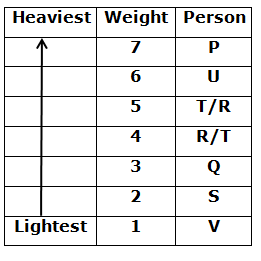ALTERNATIVE

BMUFSOBUJWF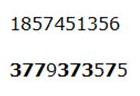Directions (6-10) :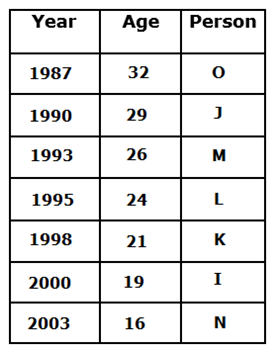Seven persons are born in different years such as 1987, 1990, 1993, 1995, 1998, 2000, and 2003. All the persons’ ages are calculated through till 2019.

• I was born in an even-numbered year but younger than J who is older than L. Difference between the ages of I and J was the same as between M and N. Both I and N are younger than M and J.
• I is not the youngest one who neither born in 2003 so the option is I was born in 2000 and N was born in 2003.
•  The difference between the ages of I and J is 10 years.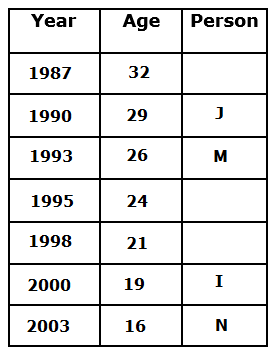Difference between the ages of I and J is double the difference between the ages of N and K. So the ages of I is 19 and J was 29 difference between the two of them is 10. Possible is J and K difference is 5. So K was born in 1998. I was born in an even-numbered year but younger than J who is older than L.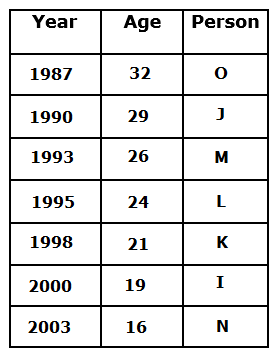### SBI PO Prelims Reasoning Day 01 Practice Set Free PDF Download

#### For Day Wise SBI PO Practice Questions

Get Reasoning Study Materials

Get All Section Study Materials

5 1 vote
Rating